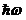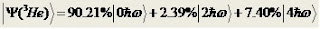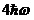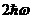## Saturday 24 August 2019

### Physics of Nucleon-Nucleus Scattering - Chapter V

Chapter Five

SPIN OBSERVABLES
IN POLARISED PROTON-HELIUM ELASTIC SCATTERING

Introduction

Elastic scattering transitions have been extensively studied over the years using the proton as a probe not only to extract information about the spin dependence of the nuclear force but also to test the validity of various theoretical models of nuclear structure and reactions.  As part of such general investigation, the few-nucleon systems, 3He and 4He, are important nuclei for theoretical and experimental studies. The prediction of the properties for the few-body nuclear system  represents a challenging test for existing theoretical models, many of which are based on various types of few-body nuclear forces, that have been developed over the years .  However, recently, the shell model approach  has been considered with such light mass systems and very good results for the structures have been obtained therefrom when large basis spaces and NN g matrix elements have been used. That has been so with proton scattering data analyses as well .

Thus we have OBDME and SP wave functions which enable us to make predictions of p-3,4He scattering by defining g-folding optical potentials. Note that with p-3He elastic scattering, there are contributions from angular momentum transfer (I) values of 0 and 1. The set of OBDME with I = 0 define the nonlocal optical potential. The I=1 contributions are small  but are included in all results  shown here. They have been evaluated as in Ref.  by using a DWA.

We consider herein just what may be achieved with that approach in analyses of 800 MeVscattering allowing minimal relativity. The process has been used
 with some success to analyzescattering data taken with 200 and 300 MeV incident proton energies. Thus the data  have been analyzed using the  structure for 3He given  in that study . The folding to give the optical potentials have been made using effective interactions built from the g matrices of the complex NN interactions  based upon the BCC3 base interaction, as  was used in my calculations of p-12C at 800 MeV.

Shell model structures of   3,4He

The structures of 3H and 3He have been one of the successes of few-body physics and of the Faddeev approach in particular. However, it is instructive to consider a shell model description of such light mass nuclei as this approach offers an alternate means to investigate the correlations in the wave functions that are naturally contained, but can be difficult to identify, in the few-body schemes. To be relevant, this description must give the basic static and reaction properties of the mass 3 nuclei in reasonable if not as good agreement with observed results. The structure studies of Navratil and Barrett  show satisfactory agreement.

The simplest shell model that may be constructed for these mass 3 nuclei is to take 3 nucleons in the 0s shell. That model however does not involve correlations in the ground state wave function that implicitly are included in the solutions of the Faddeev equations. Such correlations can be inherent in the shell model wave functions but only from shell model calculations made with much larger model spaces. Even so, convergence with basis size on some properties is slow. For example, from calculations of the ground state of the 3He performed in a shell model including  up to 32excitations , the binding energy is still a few percent away from the exact value given by the Faddeev solution for the specified NN interaction.

However, the binding energy reflects the large distance properties of the ground state wave function. On the other hand, and for the momentum transfer values usually involved, most scattering processes are sensitive to details of the wave functions within the body of the nucleus. Hence the interests in using a large space shell model wave function in calculations of the elastic scattering of electrons and protons from 3He.

Dortmans et al.  defined wave functions for the ground states of 3He and 4He within a completeshell model using the G-matrix interaction of Zheng et al. . The shell model code OXBASH   was used to obtain those wave functions. From that study the wave function of the ground state of 3He segments as,

and that  of the ground state of 4He segments as

Although both nuclei are dominantlyin nature, the significant mixing of highercomponents is expected as both systems are highly correlated. The calculated binding energies are -6.763 and -25.549 MeV for 3He and 4He respectively, which are to be compared with the measured values of -7.718 and -28.296 MeV [173, 174]. It is this stronger binding in 4He which is responsible for the enhanced mixing of higher  components. These binding energy calculations are of a similar accuracy to those made using  the   few body techniques [173-175]. The lack of convergence in the binding energies with this wave function is demonstrated in the strength of thecomponent relative to that of theone. But the essential aspect is that this wave function well describes the matter properties of 3He to a radius of import for electron and, at lower energies, proton elastic scattering .

The Zheng interaction has a unique property not associated with the usual fitted (phenomenological) interactions. It is defined by the G-matrix elements of a realistic NN potential which required specification of HO SP wave functions at the outset. Thus, in principle, there are no parameters left for adjustment in making analyses of electron and proton scattering observables. The longitudinal form factor for elastic scattering of electrons found using the specified SP wave functions  is almost an exact reproduction of the data. But the transverse (magnetic) form factor for electron scattering from 3He was underpredicted by an order of magnitude. However, this magnetic form factor is a small magnitude quantity.

Analyses of proton scattering data from 3He at 200 and 300 MeV was also made in that study . Such analyses required the additional specification of the (complex and nonlocal) optical potential, which was derived from the self same g-folding approach that has been adopted herein. That description then was also parameter free, and the predictions for the 200 and 300 MeV proton scattering observables  agreed well with data . Those results encourage credence in use of many-body methods to give a satisfactory description of 3He within at least a sphere of a few fermi radius,  so long as the structure model is defined by a large basis space model.

Results and discussion

Results from 3He

The differential cross section, analyzing power, and the spin transfer observables forelastic scattering at 800 MeV proton incident energy are compared with   experimental results in Figs. 5.1, 5.2, and 5.3.

Figure 5.1: The differential cross sections (top), and analyzing powers (bottom) from the elastic scattering of 800 MeV protons from 3He are compared with the results found using the g-folding optical potential (solid curves). Data were taken from Ref. .

Figure 5.2: The spin observables, DNN (top)  and  DLL (bottom)  from the elastic scattering of 800 MeV protons from 3He are compared with the results found using the g-folding optical potential (solid curves). Data were taken from .

In Fig. 5.1, the differential cross section and analyzing power are compared with the results found using the g-folding optical potential. Those predictions are displayed by the solid curves. The cross section and analyzing power data span a scattering angle range to 50o in the center of mass, with the cross section values from 30o onwards being of the order of 0.1 mb/sr.

Dashed vertical lines are given in each plot to note where the cross section has become that small. This indicates where the model calculation may break down. In comparison to measured values of the cross section it does.

Small changes in calculated phase shifts as would be wrought by the uncertainties in specification of the nonlocal optical potential as well as by what one could expect (or hope) from higher order contributions, will affect the results at the larger scattering angles where the scattering theory and/or the approximation inherent with its implementation are not well defined. This may be defined as the ``credibility limit" of the theoretical analysis method used.  To that angle the predicted cross section agrees very well with the data. The calculated analyzing power agrees well with the data to 25o and trends towards the minimum at 30o without reaching a null value.

Figure 5.3: The spin observables, DSL (top), DLS (middle)  and  DLL  (bottom)  from the elastic scattering of 800 MeV protons from 3He are compared with the results found using the g-folding optical potential (solid curves).

The spin transfer observables, DNN, DLL, DSL, DLS and DSS are compared with the results of calculations in Figs. 5.2 and 5.3. Over the range to 30o the experimental data for the spin transfer observable, Dnn, is practically 1.0 and the theoretical calculations reproduce this behavior. Again the theory credibility limit (at 30o) is shown in each panel by the vertical dashed lines. To that limit, the variations in measured spin observable values are quite well reproduced by the results of calculation. In particular DLL is very well defined; the calculated values of both DLS and DSL are a little too large in magnitude, while that of DSS is a bit too small.

Results from 4He

Predictions  of the   differential cross sections  and analyzing powers from the scattering of 800  MeV polarized protons from 4He are given in Fig. 5.4 where they are compared with the experimental data of Courant et al. .

The differential cross sections are well reproduced to 25o scattering angle. At the larger scattering angles the cross section values are the order of 0.1 mb/sr. Analyzing powers are not well reproduced. The trend up to 40o scattering angle is similar to the data but calculation failed to reproduce the minima.

The spin transfer observables DLL, DLS, DSL and DSS are compared with the results of calculations in Fig. 5.5 and Fig. 5.6. Data were taken from Ref. .

Clearly the general shapes of all the spin observables are described by the results of those calculations but there are some problems with the magnitudes. Since the spin observables are dependent on the differential cross sections, and for 4He the   elastic 800 MeV proton scattering cross sections are not as well reproduced by calculation as one would like, the predicted spin observables are affected and it is not surprising that fits are not as good as expected.

Figure 5.4: The differential cross sections (top), and analyzing powers (bottom) from the elastic scattering of 800 MeV protons from 4He are compared with the results found using the g-folding optical potential (solid curves). Data were taken from Refs..

Figure 5.5: The spin observables DLL (top)  and  DLS  (bottom)  from the elastic scattering of 800 MeV protons from 4He are compared with the results found using the g-folding optical potential (solid curves).

Figure 5.6: The spin observables DSL (top)  and  DSS  (bottom)  from the elastic scattering of 800 MeV protons from 4He are compared with the results found using the g-folding optical potential (solid curves).

Conclusions

In summary, the BCC3 boson exchange model NN interaction modulated have been used here by NN optical potentials with which the SM97 NN scattering phase shifts to 2.5 GeV were reproduced to specify NN t- and g-matrices at 800 MeV.

Coordinate space effective interaction forms that map those t- and g-matrices have been determined and then used in a g-folding process to specify a complex and nonlocal optical potential for 800 MeV protons incident on 3,4He.

The structure of the target used in that folding was determined from a large space shell model calculation; the ground state wave function of which leads to an electron scattering longitudinal form factor in good agreement with measured values. Thereby all quantities required in the folding process have been preset to make solution of the associated nonlocal p-3,4He Schrodinger equations predictive of the scattering phase shifts, and so of the differential cross sections and spin transfer observables. Most predicted results agree quite well with the observation for momentum transfer values to that at which the cross section is of the order of 0.1 mb/sr.